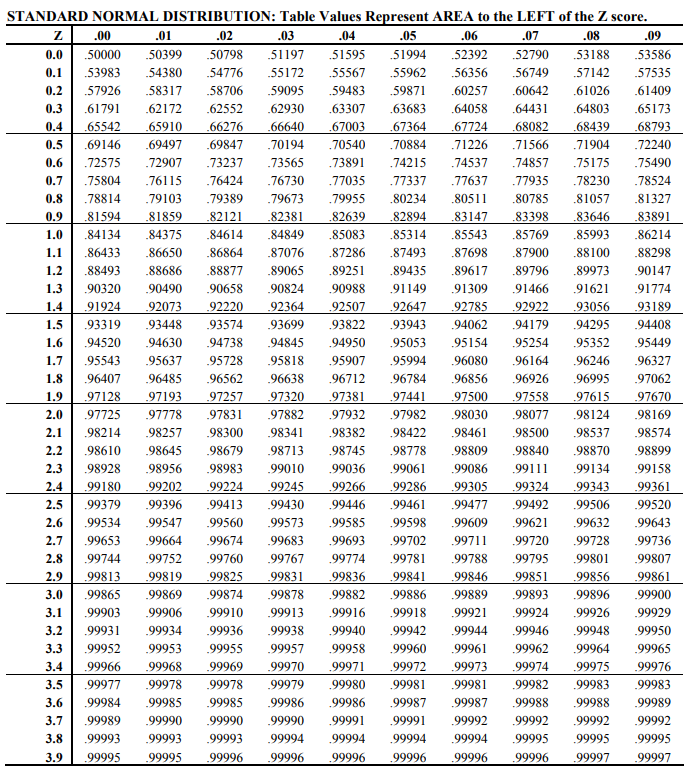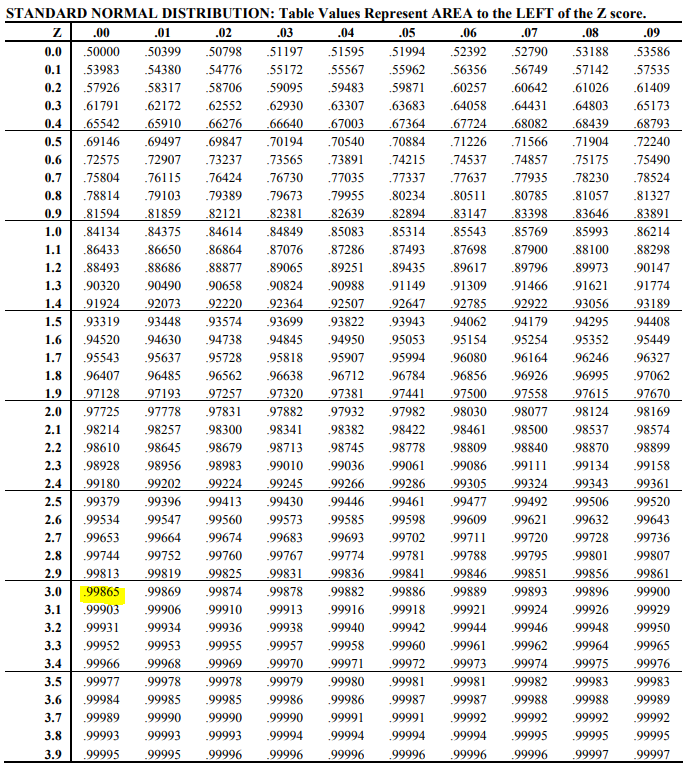## Obstacle Course

Suppose a group of students are running through a timed obstacle course for class. Their recorded times for course completion form a normal distribution with a mean of 8.0 minutes and a standard deviation of 2.5 minutes. What is the probability that a student (selected at random) takes longer than 15.5 minutes to finish the course? Refer to the below Unit Normal Distribution table.Hint
$$Z=\frac{x-\mu }{\sigma }$$$Mean $$\mu=8$$ Standard Deviation $$\sigma=2.5$$ $$x=15.5$$$
$$Z=\frac{x-\mu }{\sigma }=\frac{15.5-8}{2.5}=3.00$$$Referring to the Unit Normal Distribution Table:Because the label for rows contains the integer part and the first decimal place of Z, and the label for columns contains the second decimal place of Z, we find that .99865 is our corresponding distribution for $$Z=3.00$$ . This represents the probability of selecting a student whose course completion time is under 15.5 minutes. To find the probability of selecting a student who takes LONGER than 15.5 minutes: $$1-.99865=0.00135\:probability$$$
$$0.00135\:probability$$\$